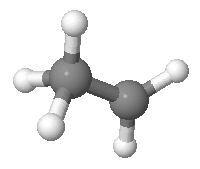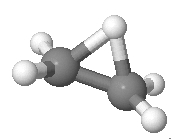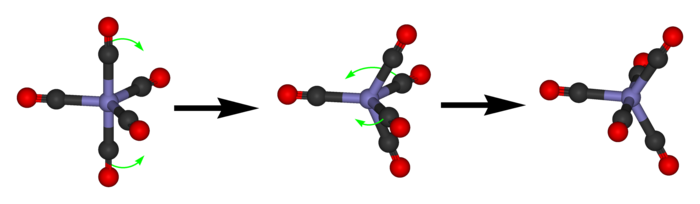# The Azimuth Project Blog - network theory (part 14)

This page is a blog article in progress, written by John Baez. To see discussions of it as it was being written, go to the Azimuth Forum. For the final polished version, visit the Azimuth Blog.

We’ve been doing a lot of hard work lately. Let’s take a break and think about a fun example from chemistry!

#### The ethyl cation

Suppose you start with a molecule of ethane, which has 2 carbons and 6 hydrogens arranged like this:

Then suppose you remove one hydrogen. The result is a positively charged ion, or ‘cation’. This particular one is called an ‘ethyl cation’. People used to think it looked like this:They also thought a hydrogen could hop from the carbon with 3 hydrogens attached to it to the carbon with 2. So, they drew a graph with a vertex for each way the hydrogens could be arranged, and an edge for each hop. It looks really cool:

The red vertices come from arrangements where the first carbon has 2 hydrogens attached to it, and the blue vertices come from those where the second carbon has 2 hydrogens attached to it. So, each edge goes between a red vertex and a blue vertex.

This graph has 20 vertices, which are arrangements or ‘states’ of the ethyl cation. It has 30 edges, which are hops or ‘transitions’. Let’s see why those numbers are right.

First I need to explain the rules of the game. The rules say that the 2 carbon atoms are distinguishable: there’s a ‘first’ one and a ‘second’ one. The 5 hydrogen atoms are also distinguishable. But, all we care about is which carbon atom each hydrogen is bonded to: we don’t care about further details of its location. And we require that 2 of the hydrogens are bonded to one carbon, and 3 to the other.

If you’re a physicist, you may wonder why the rules work this way: after all, at a fundamental level, identical particles aren’t really distinguishable. I’m afraid I can’t give a fully convincing explanation right now: I’m just reporting the rules as they were told to me!

Given these rules, there are 2 choices of which carbon has two hydrogens attached to it. Then there are $\binom{5}{2} = 10$ choices of which two hydrogens are attached to it. This gives a total of $2 \times 10 = 20$ states. These are the vertices of our graph: 10 red and 10 blue.

The edges of the graph are transitions between states. The idea is that any hydrogen in the group of 3 can hop over to the group of 2. There are 3 choices for which hydrogen atom makes the jump. So, starting from any vertex in the graph there are 3 edges. This means there are $3 \times 20 / 2 = 30$ edges. Why divide by 2? Because there are 3 edges touching each of 20 vertices, but each edge actually touches 2 vertices, so we must divide by 2 to avoid double-counting them.

#### The Desargues graph

The idea of using this graph in chemistry goes back to this paper:

• A. T. Balaban, D. Fǎrcaşiu and R. Bǎnicǎ, Graphs of multiple 1,2-shifts in carbonium ions and related systems, Rev. Roum. Chim. 11 (1966), 1205.

This paper is famous because it was the first to use graphs in chemistry to describe molecular transitions, as opposed to using them as pictures of molecules!

But this particular graph was already famous for other reasons. It’s called the Desargues-Levi graph, or Desargues graph for short:

Desargues graph, Wikipedia.

Later I’ll say why it’s called this.

There are lots of nice ways to draw the Desargues graph. For example:

The reason why we can draw such pretty pictures is that the Desargues graph is very symmetrical. Clearly any permutation of the 5 hydrogens acts as a symmetry of the graph, and so does any permutation of the 2 carbons. This gives a symmetry group $S_5 \times S_2$, which has $5! \times 2! = 240$ elements. And in fact this turns out to be the full symmetry group of the Desargues graph.

The Desargues graph, its symmetry group, and its applications to chemistry are discussed here:

• Milan Randić, Symmetry properties of graphs of interest in chemistry: II: Desargues-Levi graph, Int. Jour. Quantum Chem. 15 (1997), 663-682.

#### The ethyl cation, revisited

We can try to describe the ethyl cation using probability theory. If at any moment its state corresponds to some vertex of the Desargues graph, and it hops randomly along edges as time goes by, it will trace out a random walk on the Desargues graph. This is a nice example of a Markov process!

We could also try to describe the ethyl cation using quantum mechanics. Then, instead of having a probability of hopping along an edge, it has an amplitude of doing so. But as we’ve seen, a lot of similar math will still apply.

It should be fun to compare the two approaches. But I bet you’re wondering which approach is correct. This is a somewhat tricky question, at least for me. The answer would seem to depend on how much the ethyl cation is interacting with its environment—for example, bouncing off other molecules. When a system is interacting a lot with its environment, a probabilistic approach seems to be more appropriate. The relevant buzzword is ‘environmentally induced decoherence’.

However, there’s something much more basic I have tell you about.

After the paper by Balaban, Fǎrcaşiu and Bǎnicǎ came out, people gradually realized that the ethyl cation doesn’t really look like the drawing I showed you! In fact it’s what chemists call ‘nonclassical’ ion. What they mean is this: its actual structure is not what you get by taking the traditional ball-and-stick model of an ethane molecule and ripping off a hydrogen. The ethyl cation really looks like this:For more details, and pictures that you can actually rotate, see:

• Stephen Bacharach, Ethyl cation, Computational Organic Chemistry.

So, if we stubbornly insist on applying the Desargues graph to realistic chemistry, we need to find some other molecule to apply it to.

#### Trigonal bipyramidal molecules

Luckily, there are lots of options! They’re called trigonal bipyramidal molecules. They look like this:

The 5 balls on the outside are called ‘ligands’: they could be atoms or bunches of atoms. In chemistry, ‘ligand’ just means something that’s stuck onto a central thing. For example, in phosphorus pentachloride the ligands are chlorine atoms, all attached to a central phosphorus atom:

It’s a colorless solid, but as you might expect, it’s pretty nasty stuff: it’s not flammable, but it reacts with water or heat to produce toxic chemicals like hydrogen chloride.

Another example is iron pentacarbonyl, where 5 carbon-oxygen ligands are attached to a central iron atom:

You can make this stuff by letting powdered iron react with carbon monoxide. It’s a straw-colored liquid with a pungent smell!

Whenever you’ve got a molecule of this shape, the ligands come in two kinds. There are the 2 ‘axial’ ones, and the 3 ‘equatorial’ ones:

And the molecule has $20$ states… but only if count the states a certain way. We have to treat all 5 ligands as distinguishable, but think of two arrangements of them as the same if we can rotate one to get the other. The trigonal bipyramid has a rotational symmetry group with 6 elements. So, there are $5! / 6 = 20$ states.

The transitions between states are devilishly tricky. They’re called pseudorotations, and they look like this:If you look very carefully, you’ll see what’s going on. First the 2 axial ligands move towards each other to become equatorial.
Now the equatorial ones are no longer in the horizontal plane: they’re in the plane facing us! Then 2 of the 3 equatorial ones swing out to become axial. This fancy dance is called the Berry pseudorotation mechanism.

To get from one state to another this way, we have to pick 2 of the 3 equatorial ligands to swing out and become axial. There are 3 choices here. So, if we draw a graph with states as vertices and transitions as edges, it will have 20 vertices and $20 \times 3 / 2 = 30$ edges. That sounds suspiciously like the Desargues graph!

Puzzle 1. Show that the graph with states of a trigonal bipyramidal molecule as vertices and pseudorotations as edges is indeed the Desargues graph.

I think this fact was first noticed here:

• Paul C. Lauterbur and Fausto Ramirez, Pseudorotation in trigonal-bipyramidal molecules, J. Am. Chem. Soc. 90 (1968), 6722–6726.

Okay, enough for now! Next time I’ll say more about the Markov process or quantum process corresponding to a random walk on the Desargues graph. But since the Berry pseudorotation mechanism is so hard to visualize, I’ll pretend that the ethyl cation looks like this:and I’ll use this picture to help us think about the Desargues graph. That’s okay: everything we’ll figure out can easily be translated to apply to the real-world situation of a trigonal bipyramidal molecule. The virtue of math is that when two situations are ‘mathematically the same’, or ‘isomorphic’, we can talk about either one, and the results automatically apply to the other. This is true even if the one we choose to talk about doesn’t actually exist in the real world!

category: blog